## Example 26.18 Fitting Direct Covariance Structures

In the section Direct Covariance Structures Analysis, the MSTRUCT modeling language is used to specify a model with direct covariance structures. In the model, four variables from the data set of Wheaton et al. (1977) are used. The analysis is carried out in this example to investigate the tenability of the hypothesized covariance structures.

The four variables used are: Anomie67, Powerless67, Anomie71, and Powerless71. The hypothesized covariance matrix is structured as: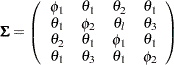where: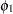:

variance of anomie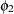:

variance of powerlessness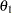:

covariance between anomie and powerlessness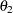:

covariance between anomie measures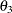:

covariance between powerlessness measures

In this example, you hypothesize the covariance structures directly, as opposed to those models with implied covariance structures from path models (see Example 26.16), structural equations (see Example 26.17), or other types of models. The basic assumption of the direct covariance structures in this example is that Anomie and Powerless were invariant over the measurement periods employed. This implies that the time of measurement did not change the variances and covariances of the measures. Therefore, both Anomie67 and Anomie71 have the same variance parameter, and both Powerless67 and Powerless71 have the same variance parameter. These two parameters,and, are hypothesized on the diagonal of the covariance matrix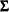. In the same structured covariance matrix,represents the covariance between Anomie and Powerless, without regard to the time of measurement. Theparameter represents the covariance between the Anomie measures, or the reliability of the Anomie measure. Similarly, theparameter represents the covariance between the Powerless measures, or the reliability of the Anomie measure.

As explained in the section Direct Covariance Structures Analysis, you can use the MSTRUCT modeling language to specify the hypothesized covariance structures directly, as shown in the following statements:

```proc calis nobs=932 data=Wheaton psummary;
fitindex on(only)=[chisq df probchi] outfit=savefit;
mstruct
var = Anomie67 Powerless67 Anomie71 Powerless71;
matrix _COV_ [1,1] = phi1,
[2,2] = phi2,
[3,3] = phi1,
[4,4] = phi2,
[2,1] = theta1,
[3,1] = theta2,
[3,2] = theta1,
[4,1] = theta1,
[4,2] = theta3,
[4,3] = theta1;
run;
```

In the MSTRUCT statement you specify the variables in the VAR= list. The order of variables in this VAR= list is assumed to be the same as that in the row and column of the hypothesized covariance matrix. Next, in the MATRIX statement you specify parameters as entries in the hypothesized covariance matrix _COV_. Only the lower diagonal elements need to be specified because covariance matrices, by nature, are symmetric. Redundant specification of the upper triangular elements are unnecessary as PROC CALIS has the information accounted for. You can also set initial estimates by putting parenthesized numbers after the parameter names. But in this example you let PROC CALIS determine all the initial estimates.

In the PROC CALIS statement, the PSUMMARY option is used. As a global display option, this option suppresses a lot of displayed output and requests only the fit summary table be printed. This way you can eliminate quite a lot of displayed output that is not of your primary interest. In this example, the specification of the covariance structures is straightforward, and you do not need any output regarding the initial estimation or standardized solution. Suppose that you are not even concerned with the estimates of the parameters because you are not yet sure if this model is good enough for the data. All you want to know at this stage is whether the hypothesized covariance structures fit the data well. Therefore, the PSUMMARY option would serve your purpose well in this example.

In fact, even the fit summary table can be trimmed down quite a bit if you only want to look at certain specific fit indices. In the FITINDEX statement of this example, the ON(ONLY)= option turns on the printing of the model fit chi-square, its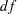, and-value only. This does not mean that you must lose the information of all other fit indices. In addition to the printed output, you can save all fit indices in an output data set. To this end, you can use the OUTFIT= option in the FITINDEX statement. In this example, you save the results of all fit indices in a SAS data set called savefit.

Output 26.18.1 shows the entire printed output.

Output 26.18.1 Testing Direct Covariance Structures
Fit Summary
Chi-Square 221.5798
Chi-Square DF 5
Pr > Chi-Square <.0001

The displayed output is very concise. It contains only a fit summary table with three statistics. The-value for the model fit chi-square test indicates that the hypothesized structures should be rejected at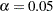. Therefore, this rather restrictive direct covariance structure model does not fit the data well. A less restrictive covariance structure model is needed to explain the variances and covariances.

All fit indices are saved in the savefit data set. To view it, you can use the following statement:

```proc print data=savefit;
run;
```

Output 26.18.2 shows all indices, their types and values of all fit indices and information.

Output 26.18.2 Saved Fit Indices
 Analysis of Direct Covariance Structures Testing Model by the MSTRUCT Language

Obs _TYPE_ IndexCode FitIndex FitValue PrintChar
1 ModelInfo 101 N Observations 932.00 932
2 ModelInfo 103 N Variables 4.00 4
3 ModelInfo 104 N Moments 10.00 10
4 ModelInfo 105 N Parameters 5.00 5
5 ModelInfo 106 N Active Constraints 0.00 0
6 ModelInfo 111 Baseline Model Function Value 1.68 1.6799
7 ModelInfo 113 Baseline Model Chi-Square 1563.94 1563.9442
8 ModelInfo 114 Baseline Model Chi-Square DF 6.00 6
9 ModelInfo 115 Pr > Baseline Model Chi-Square 0.00 <.0001
10 Absolute 201 Fit Function 0.24 0.2380
11 Absolute 203 Chi-Square 221.58 221.5798
12 Absolute 204 Chi-Square DF 5.00 5
13 Absolute 205 Pr > Chi-Square 0.00 <.0001
14 Absolute 211 Z-Test of Wilson & Hilferty 12.25 12.2533
15 Absolute 212 Hoelter Critical N 47.00 47
16 Absolute 213 Root Mean Square Residual (RMSR) 0.76 0.7649
17 Absolute 214 Standardized RMSR (SRMSR) 0.07 0.0701
18 Absolute 215 Goodness of Fit Index (GFI) 0.90 0.9036
19 Parsimony 301 Adjusted GFI (AGFI) 0.81 0.8071
20 Parsimony 302 Parsimonious GFI 0.75 0.7530
21 Parsimony 303 RMSEA Estimate 0.22 0.2157
22 Parsimony 304 RMSEA Lower 90% Confidence Limit 0.19 0.1920
23 Parsimony 305 RMSEA Upper 90% Confidence Limit 0.24 0.2404
24 Parsimony 306 Probability of Close Fit 0.00 <.0001
25 Parsimony 307 ECVI Estimate 0.25 0.2488
26 Parsimony 308 ECVI Lower 90% Confidence Limit 0.20 0.2003
27 Parsimony 309 ECVI Upper 90% Confidence Limit 0.31 0.3053
28 Parsimony 310 Akaike Information Criterion 231.58 231.5798
29 Parsimony 311 Bozdogan CAIC 260.77 260.7665
30 Parsimony 312 Schwarz Bayesian Criterion 255.77 255.7665
31 Parsimony 313 McDonald Centrality 0.89 0.8903
32 Incremental 401 Bentler Comparative Fit Index 0.86 0.8610
33 Incremental 402 Bentler-Bonett NFI 0.86 0.8583
34 Incremental 403 Bentler-Bonett Non-normed Index 0.83 0.8332
35 Incremental 404 Bollen Normed Index Rho1 0.83 0.8300
36 Incremental 405 Bollen Non-normed Index Delta2 0.86 0.8611
37 Incremental 406 James et al. Parsimonious NFI 0.72 0.7153

The results of various fit indices from this output data set confirm that the hypothesized model does not fit the data well.

As an aside, it is noted with some shorthand notation, the specification of the MSTRUCT model parameters that use the MATRIX statements can be made a little more precise for the current example. This is shown as follows:

```proc calis nobs=932 data=Wheaton psummary;
mstruct
var = Anomie67 Powerless67 Anomie71 Powerless71;
matrix _COV_ [1,1] = phi1 phi2 phi1 phi2,
[2, ] = theta1,
[3, ] = theta2 theta1,
[4, ] = theta1 theta3 theta1;
fitindex on(only)=[chisq df probchi] outfit=savefit;
run;
```

In the first entry of the MATRIX statement, the notation [1,1] represents that the parameter list specified after the equal sign starts with the [1,1] element of the _COV_ matrix and proceeds down the diagonal. In the next three entries, the notations [2,], [3,], and [4,] represent that parameter lists start with the first elements of the second, third, and fourth rows, respectively, and proceed to the next (right) elements on the same rows. See the syntax of the MATRIX statement for more details about this kind of shorthand notation.

This example shows how you can use the MSTRUCT modeling language to test specific covariance patterns. You need to define the parameters of the covariance patterns explicitly by the MATRIX statements. See Example 26.4 and Example 26.20 for more applications.

However, some commonly-used covariance and mean patterns are built into PROC CALIS. For these covariance and mean patterns, you can simply use the COVPATTERN= and the MEANPATTERN= options without the need to specify the parameters in the MATRIX statements. See the COVPATTERN= and the MEANPATTERN= options for the supported covariance and mean patterns. See Example 26.5 and Example 26.21 for applications.